# Python Array Slicing Damian Gordon Array Slicing Array

• Slides: 17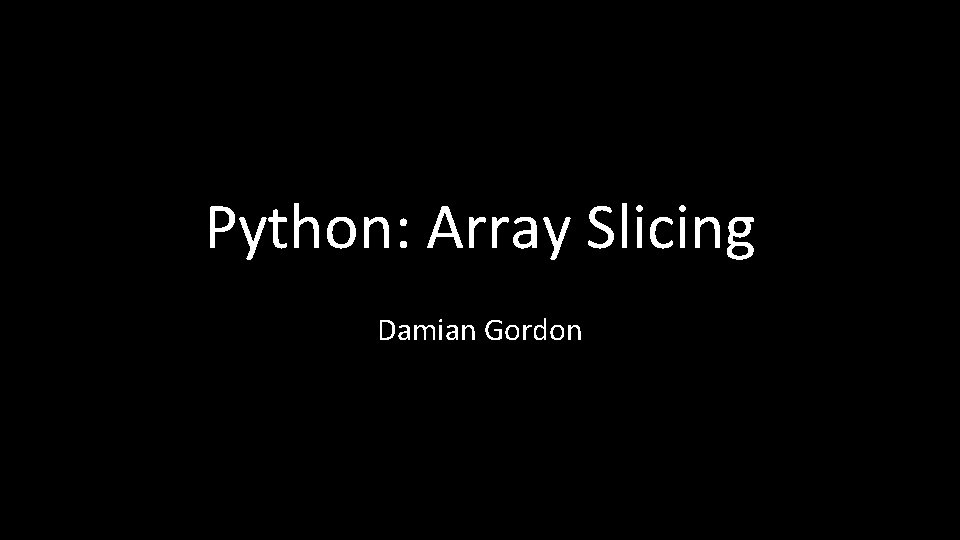Python: Array Slicing Damian GordonArray Slicing • Array Slicing is a way of quickly printing out sections of an array. • It is not unique to Python, and is available in other languages such as Fortran, Algol, Ada, and MATLAB.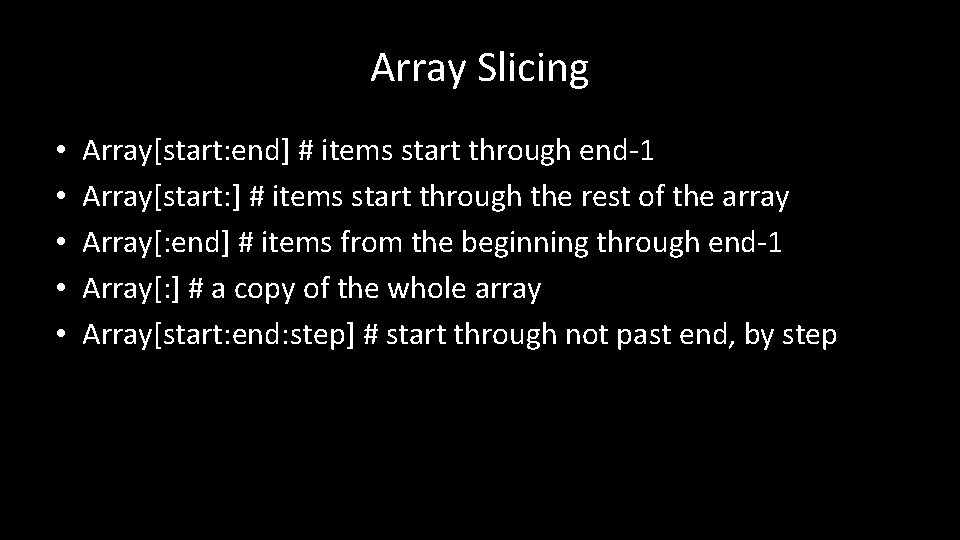Array Slicing • • • Array[start: end] # items start through end-1 Array[start: ] # items start through the rest of the array Array[: end] # items from the beginning through end-1 Array[: ] # a copy of the whole array Array[start: end: step] # start through not past end, by step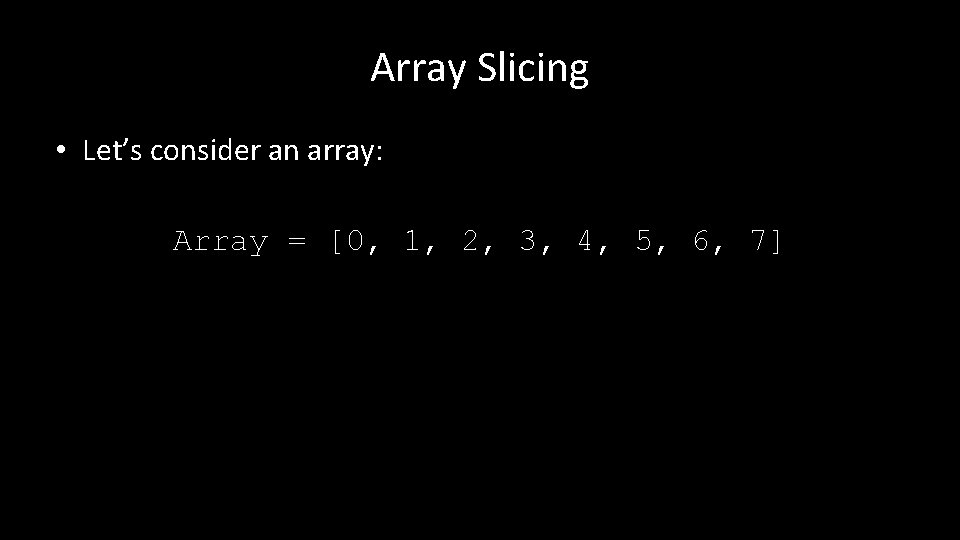Array Slicing • Let’s consider an array: Array = [0, 1, 2, 3, 4, 5, 6, 7]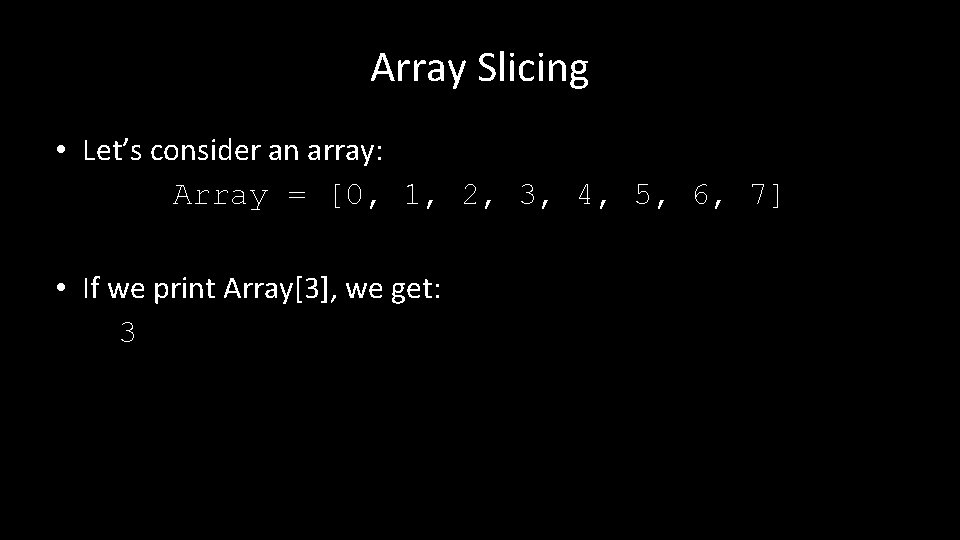Array Slicing • Let’s consider an array: Array = [0, 1, 2, 3, 4, 5, 6, 7] • If we print Array, we get: 3Array Slicing • Let’s consider an array: Array = [0, 1, 2, 3, 4, 5, 6, 7] • If we print Array[: 3], we get: [0, 1, 2]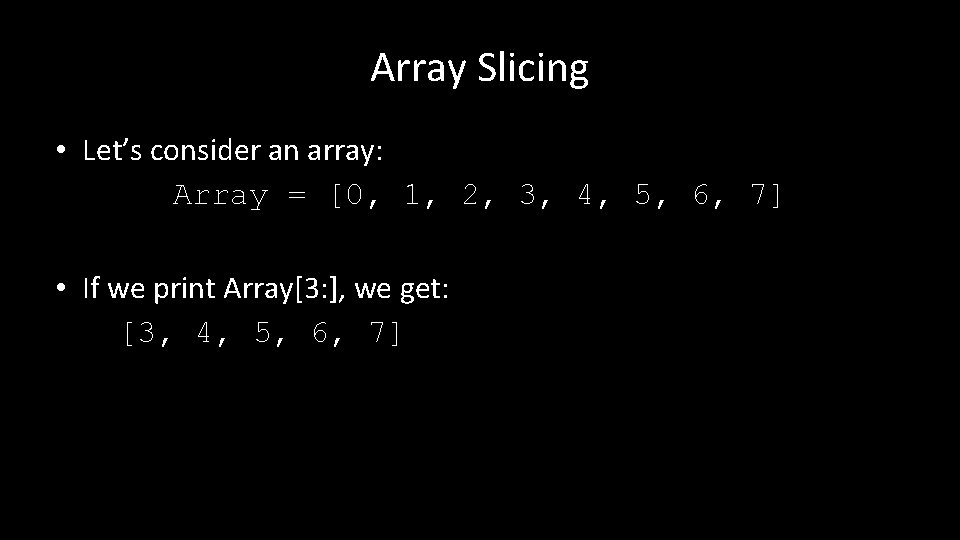Array Slicing • Let’s consider an array: Array = [0, 1, 2, 3, 4, 5, 6, 7] • If we print Array[3: ], we get: [3, 4, 5, 6, 7]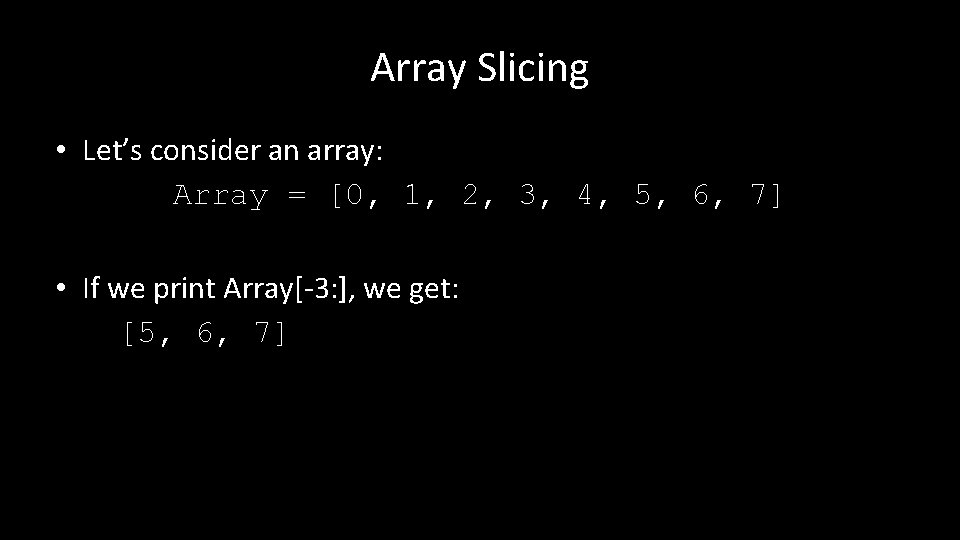Array Slicing • Let’s consider an array: Array = [0, 1, 2, 3, 4, 5, 6, 7] • If we print Array[-3: ], we get: [5, 6, 7]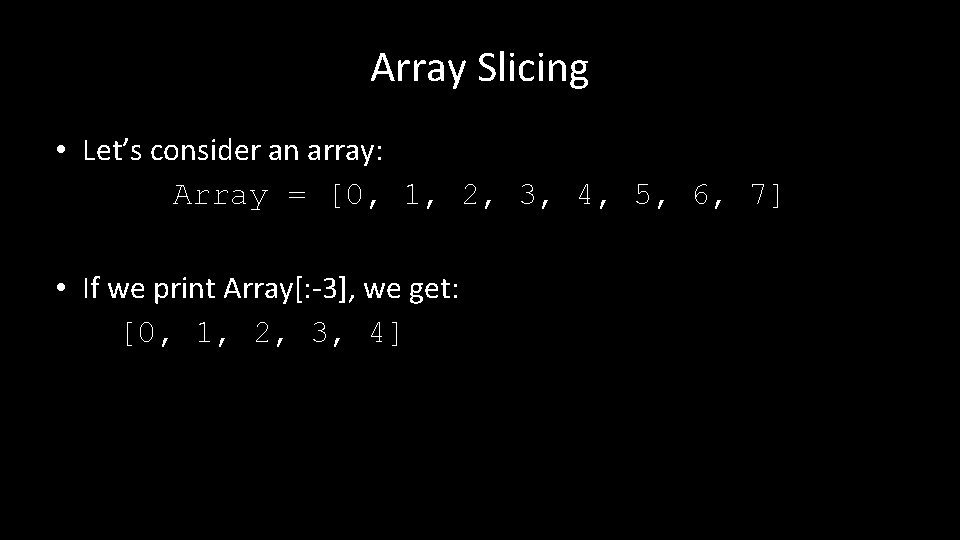Array Slicing • Let’s consider an array: Array = [0, 1, 2, 3, 4, 5, 6, 7] • If we print Array[: -3], we get: [0, 1, 2, 3, 4]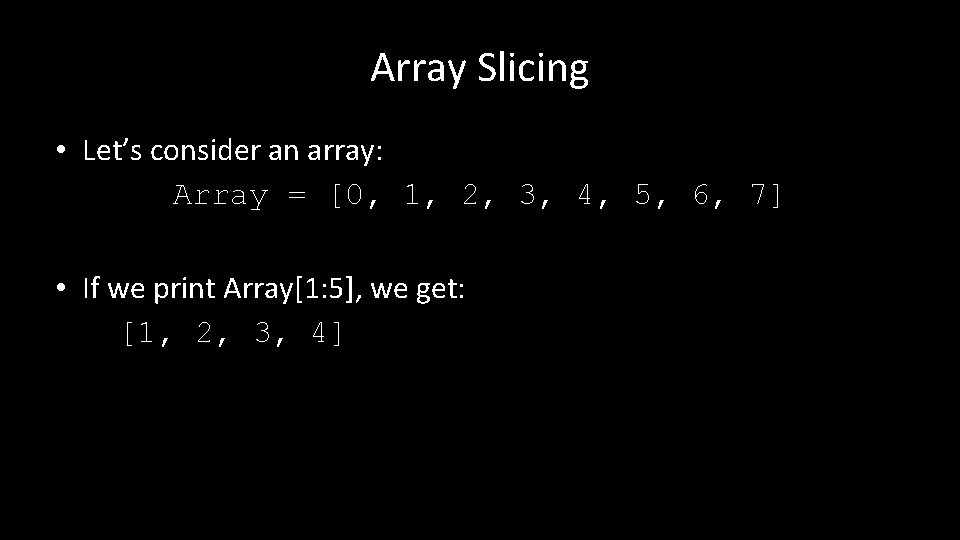Array Slicing • Let’s consider an array: Array = [0, 1, 2, 3, 4, 5, 6, 7] • If we print Array[1: 5], we get: [1, 2, 3, 4]Array Slicing • Let’s consider an array: Array = [0, 1, 2, 3, 4, 5, 6, 7] • If we print Array[1: 5: 2], we get: [1, 3]Array Slicing • Let’s try a 2 D Array: MArray = [[0, 1, 2], [3, 4, 5], [6, 7, 8]]Array Slicing • Let’s try a 2 D Array: MArray = [[0, 1, 2], [3, 4, 5], [6, 7, 8]] • Let’s print MArray[1: ] [[3, 4, 5], [6, 7, 8]]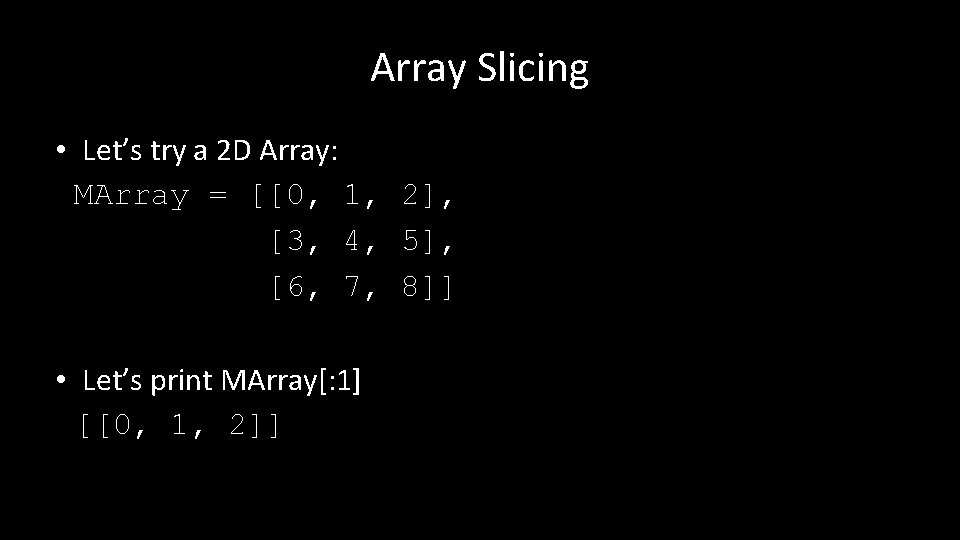Array Slicing • Let’s try a 2 D Array: MArray = [[0, 1, 2], [3, 4, 5], [6, 7, 8]] • Let’s print MArray[: 1] [[0, 1, 2]]Array Slicing • Let’s try a 2 D Array: MArray = [[0, 1, 2], [3, 4, 5], [6, 7, 8]] • Let’s print MArray[1: 2] [[3, 4, 5]]Array Slicing • Let’s try a 2 D Array: MArray = [[0, 1, 2], [3, 4, 5], [6, 7, 8]] • Let’s print MArray[2: 3] [[6, 7, 8]]etc.